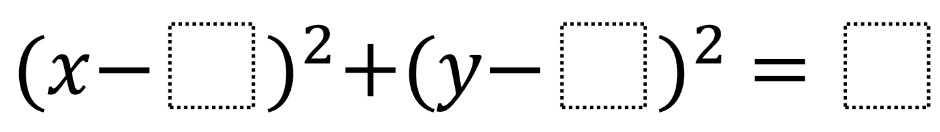# Circle Tangent to Line

Directions: Using the digits 0 to 9 at most one time each, place a digit in each box to create a circle tangent to the line x+y=5.### Hint

What is the slope of the tangent line? How must that slope relate to the radius of the circle?